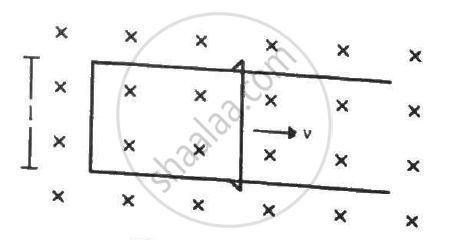Advertisement Remove all ads

# Figure Shows a Long U-shaped Wire of Width L Placed in a Perpendicular Magnetic Field B. - Physics

Sum

Figure shows a long U-shaped wire of width l placed in a perpendicular magnetic field B. A wire of length l is slid on the U-shaped wire with a constant velocity v towards right. The resistance of all the wires is r per unit length. At t = 0, the sliding wire is close to the left edge of the U-shaped wire. (a) Calculate the force needed to keep the sliding wire moving with a constant velocity v. (b) If the force needed just after t = 0 is F0, find the time at which the force needed will be F0/2.0Advertisement Remove all ads

#### Solution

Emf induced in the circuit, e = Bvl

Current in the circuit,

$i = \frac{e}{R} = \frac{Bvl}{2r(l + vt)}$

(a) Force F needed to keep the sliding wire moving with a constant velocity v will be equal in magnitude to the magnetic force on it. The direction of force F will be along the direction of motion of the sliding wire.

Thus, the magnitude of force F is given by

$F = ilB = \frac{Bvl}{2r(l + vt)} \times lB$

$= \frac{B^2 l^2 v}{2r(l + vt)}$

(b) The magnitude of force F at t = 0 is given by

$F_0 = ilB = lB\left( \frac{lBv}{2rl} \right)$

$= \frac{l B^2 v}{2r} .............(1)$

Let at time t = T,  the value of the force be F0/2.

Now,

$\frac{F_0}{2} = \frac{l^2 B^2 v}{2r(l + vT)}$

On substituting the value of F0 from (1), we get

$\frac{l B^2 v}{4r} = \frac{l^2 B^2 v}{2r(l + vT)}$

$\Rightarrow 2l = l + vT$

$\Rightarrow T = \frac{l}{v}$

Is there an error in this question or solution?
Advertisement Remove all ads

#### APPEARS IN

HC Verma Class 11, Class 12 Concepts of Physics Vol. 2
Chapter 16 Electromagnetic Induction
Q 37 | Page 308
Advertisement Remove all ads

#### Video TutorialsVIEW ALL 

Advertisement Remove all ads
Share
Notifications

View all notifications

Forgot password?